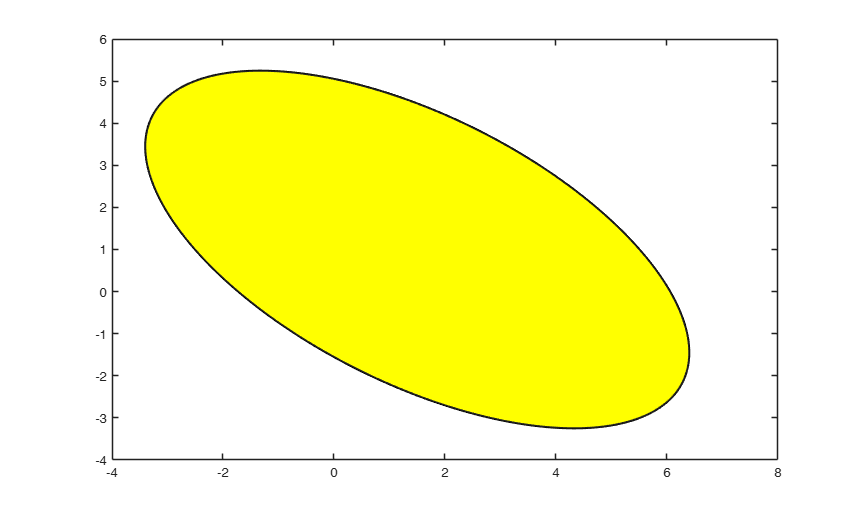# ellipse

ellipse generates an ellipse given mu (location vector) and Sigma (scatter matrix)

## Syntax

• Ell =ellipse(mu, Sigma)example
• Ell =ellipse(mu, Sigma, conflev)example
• Ell =ellipse(mu, Sigma, conflev, Color)example
• Ell =ellipse(mu, Sigma, conflev, Color, axesellipse)example
• [Ell , he]=ellipse(___)example

## Description

The ellipse is generated using the equation:

$(x-\mu)' \Sigma^{-1} (x-\mu) = c_{conflev}^2.$ The length of the i-th principal semiaxis $(i=1, 2)$ is $c_{conflev} \sqrt \lambda_i$ where $\lambda_i$ is the $i$-th eigenvalue of $\Sigma$.

 Ell =ellipse(mu, Sigma) Example using all default options.

 Ell =ellipse(mu, Sigma, conflev) Draw the ellipse using a blue color line.

 Ell =ellipse(mu, Sigma, conflev, Color) Draw an ellipse and fill it with yellow color.

 Ell =ellipse(mu, Sigma, conflev, Color, axesellipse) 99 per cent confidence ellipse.

 [Ell , he] =ellipse(___) 99 per cent confidence ellipse without showing the major axes.

## Examples

expand all

### Example using all default options.

rho=-2;
A=[4 rho; rho 3 ];
mu=[1.5 1];
ellipse(mu,A);

###Draw the ellipse using a blue color line.

close all
rho=-2;
A=[4 rho; rho 3 ];
mu=[1.5 1];
Color=[0 0 1];
ellipse(mu,A,[],Color);###Draw an ellipse and fill it with yellow color.

close all
rho=-2;
A=[4 rho; rho 3 ];
mu=[1.5 1];
Ell=ellipse(mu,A);
patch(Ell(:,1),Ell(:,2),'y');###99 per cent confidence ellipse.

Generate 1000 bivariate normal data and add the ellipse which contains approximately 990 of them.

rng('default')
rng(20)     % For reproducibility
% Define mu and Sigma
mu = [2,3];
Sigma = [1,1.5;1.5,3];
Y = mvnrnd(mu,Sigma,1000);
figure
hold on;
plot(Y(:,1),Y(:,2),'o');
% add an ellipse to these points
Ell=ellipse(mu,Sigma,0.99);
axis equal
% Count number of points inside the ellipse
disp('Number of points inside the ellipse')
disp(sum(inpolygon(Y(:,1),Y(:,2),Ell(:,1),Ell(:,2))))
Number of points inside the ellipse
987### 99 per cent confidence ellipse without showing the major axes.

First close all previous plots

close all
% Generate 1000 bivariate normal data and add the ellipse which
% contains approximately 990 of them.
rng('default')
rng(20)     % For reproducibility
% Define mu and Sigma
mu = [2,3];
Sigma = [1,1.5;1.5,3];
Y = mvnrnd(mu,Sigma,1000);
figure
hold on;
plot(Y(:,1),Y(:,2),'o');
% add an ellipse to these points and do not show the major axes
AxesEllipse = false;
Ell=ellipse(mu,Sigma,0.99,[],AxesEllipse);
axis equal
% Count number of points inside the ellipse
disp('Number of points inside the ellipse')
disp(sum(inpolygon(Y(:,1),Y(:,2),Ell(:,1),Ell(:,2))))

## Input Arguments

### mu — Center of the ellipse. Vector.

Vector with two elements associated with the center of the ellipse

Data Types: single| double

### Sigma — 2 x 2 symmetric positive definite matrix. Matrix.

Inverse of the matrix of the quadratic form which defines the equation of the ellipse. Sigma is interpretable as the covariance matrix of the original data points.

Data Types: single| double

### conflev — Confidence level. Positive scalar.

If conflev is a empty (default) the confidence level which controls the size of the ellipse (c_{conflev}^2= is computed as chi2inv(0.95,2). If the data are normally distributed the ellipse covers 95 per cent of the observations. If conflev is a scalar smaller than 1, the confidence level $c_{conflev}^2$ is computed as chi2inv(conflev,2). If conflev is a scalar greater or equal than 1 no chi2inv is applied to conflev and $c_{conflev}^2$=conflev.

Example: 'conflev', 0.99 

Data Types: single | double

### Color — LineColor of the ellipse. String or 3 elements numeric vector.

Line color, specified as an RGB triplet, a color string, or 'none'. If you specify the Color as 'none', then the line is invisible.

An RGB triplet is a three-element row vector whose elements specify the intensities of the red, green, and blue components of the color. The intensities must be in the range [0,1], for example, [0.4 0.6 0.7].

Example: 'Color', 'r' 

Data Types: [0 0 1] (default) | RGB triplet | color string | 'none'

### axesellipse — axes of the ellipse. Boolean.

If axes is true (default) dottted lines along the major axes of the ellipse are drawn else just the ellipse contour appears.

Example: 'axesellipse', false 

Data Types: Boolean

## Output Arguments

### Ell —x and y coordinates of the ellipse.  Matrix

630-by-2 matrix containing the x and y coordinate of the ellipse.

1st column = x coordinates;

2nd column = y coordinates.

### he —Vector of chart line objects. matlab.graphics.chart.primitive.Line

A column vector of chart line objects. It can be used to modify properties of a specific chart line of the plot containing the ellipse after it is created. For a list of properties, see Chart Line Properties.

Mardia, K.V., J.T. Kent, and J.M. Bibby (1979), "Multivariate Analysis," Academic Press, London, p. 140. [MKB].